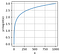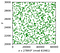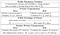# Discrete logarithm problem and Diffie-Hellman key exchange

In the previous post we learned what are ciphers and how can two parties establish a secure communication when they both hold the same secret key, for instance using a stream cipher. In this post we will see how they can agree on a common key through an insecure channel. You can find all my code for this post here.

I hope that in the previous post I convinced you that stream ciphers are good practical ciphers (used in real cryptographic applications like mobile phone communications). For the stream cipher to be secure we require that Alice and Bob agree on a cryptographically secure pseudo-random generator algorithm (CPRG) and a private key that act as a seed for the CPRG. Stream ciphers are just one class of ciphers from a broader family called symmetric ciphers (check chapters 3 to 6 from the book of Katz and Lindell where they explain private key cryptography or symmetric cryptography) whose common characteristic is that the two communicating parties Alice and Bob hold the same private key that is used for both encryption and decryption of the message.

Ok, so now we have a plethora of symmetric ciphers that one can use to communicate securely with another party. The only missing ingredient is how can Alice and Bob agree on a specific secret key (a string of bits or equivalently an integer) when communicating through a completely insecure channel. Let me reformulate this in a more mundane example. Imagine that Alice and Bob want to establish a common secret key, and they are located on opposite sides of a room full of people (the eavesdroppers). They can just communicate shouting out loud integer numbers through the room so Charlie (some random person that is in the middle of the room) can hear everything they say. Surprisingly Alice and Bob can agree on a common secret key even if Charlie is listening to all of their messages. If you you want to know how? Just keep on reading.

# The discrete logarithm problem

Warning!. For this section, you may need to refresh an older post on algebra and modulo arithmetics from this series.

You may know logarithms from your math class at school. They are pretty simple. The function logarithm is normally represented as

where x, y and b are related by

here b is known as the base of the logarithm. The logarithm problem is the problem of finding y knowing b and x, i.e. calculate the logarithm of x base b. This is super straight forward to do if we work in the algebraic field of real numbers, just have a look at the logarithm graph for base 10:logarithm base 10 as a function of x.

Nice graph, smooth, derivable… Now if I give you the following values of y=[10, 100, 1000] could you compute x knowing that b is 10? Pretty easy, those are [1, 2, 3]. If we pick values in the middle like for instance 1.5 we just need to apply simple numerical methods knowing that the function is smooth and derivable but at first glance you can guess the value is between 1 and 2.

The logarithm problem can be reformulated when instead of working in the real numbers field we work on the prime modulo algebraic field (now that you know what is a prime modulo field and how to generate large prime numbers). Specifically, we will only use the algebraic group part of the second operation, the multiplication. This is, the elements {1, 2, 3, … p-1} where p is a prime number with the operation multiplication modulo p.

The discrete logarithm problem (DLP) reads: Find y knowing x, g and p such that:

where p is a prime number and g is what we know as a generator of the group defined by p. The generator is simply an element of the field such that by powering it from 1 all the way to p-1 it generates all the elements of the group, thus {g, g², g³,…, g^(p-1)}(mod p) are all the elements of the group. Not all the elements of the group are generators and we won’t discuss here how to find them (you can find the algorithm in A Computational Introduction to Number Theory and Algebra by Victor Shoup and my implementation in Python can be found here). We’ll get to the point on why we use a generator for exponentiation later on.

Let’s take an example with (g, p) = (27893, 61981) and plot only in the for the ranges of y in between 2000 and 3000 for better visualisation.Plot of x for several values of y for the group defined by p=61981 using the group generator element g=27893.

First, a takehome exercice!. I don’t want you to blindly believe that g=27893 is a generator, just try it yourself!. Calculate all the powers of 27893 modulo 61981 and check that you generate all the numbers between 1 and p-1. Write a simple program on that to verify.

Now, let’s get back to the problem. If I give you x=53434, g=27893 and p=61981, could you guess from the graph above what’s the value of y? (spoiler, its y=29 but I bet you can’t tell). This is difficult to see as there’s no apparent pattern here compared to the field of real numbers seen previously. There’s no way also to calculate numerically the solution as before. This is a really hard problem to solve if p is a large prime (more on that in the following section).

Now, why we use a generator g instead of any number between 1 and p-1? It’s because with g we increment the search space, i.e. a priori any number between 1 and p-1 is a valid candidate for y, the number we are looking for. This makes the problem harder.

# How hard it is to break the discrete logarithm problem?

I hope I have convinced you that finding y in the DLP is a difficult task. But how hard is it?. Let’s try to break the DLP problem by randomly guessing the values of y. This is, given the triplet (x, g, p) we will randomly try different y until we find that x=g^y(mod p).

In this notebook first we generate a random prime number p of n bits and a corresponding generator g. Then randomly pick a value x in between 0 and p-1 (the secret key). At this point the protocol is set up, now the attack is to try to guess y by randomly sampling values in between 0 and p-1 and compute g^y(mod p). If this value is equal to x, then we would have cracked the DLP. On my computer (a regular laptop) I came up with the following values:

In the above table the first column is the number of bits of the random prime number generated, the second the minimum (best case scenario) search space ad the third column the computation time it took to crack the DLP in just one round (for more accurate numbers we ideally should run each case several times and make the average). Recall how fast the difficulty increases, for instance when increasing from 20 to 21 bits we go from 7 seconds to 15 seconds, and that’s a huge increase!. Try it yourself for 32 bits here, see how long can you wait before aborting the calculation.

When working with computationally hard problems to solve one can use The concrete approach definition for computational security (see An introduction to modern cryptography): A scheme is (t, e) secure if any adversary running at most during time t succeeds to break the code within probability at most e. Say a supercomputer can do c key test per unit time, then at time t he will have run c*t checks. The probability of guessing the key of length n bits is

This probability has to be smaller than e so that the scheme is (t, e) secure. Recall that as the size of the key (n) grows the probability decreases exponentially so comparing we need a lot of time (because it grows linearly) to get to the same probability.

Just to mention, there’s another approach to computational security that instead of dealing with times it deals with complexity of algorithms. That’s the asymptotic approach and you can learn more formally about it in this book.

# The Diffie-Hellman key exchange

Now let’s get back to the main aim of this post, that is, to find a way for Alice and Bob to agree on a number when communicating through an insecure channel. For this we can exploit the difficulty to crack the DLP problem (see the book of Hoffstein, Pipher and Silverman). In the book you find a nice chart explaining the Diffie-Hellman key exchange:Diffie-Hellman key exchange. Table 2.2 from the book of Hoffstein Pipher and Silverman. A masterpiece, you must buy it!

First Alice and Bob agree on a prime number p and a generator g of the group of integers mod p. This is something public and any eavesdropper knows it. Then Alice and Bob secretly choose two random numbers a and b respectively in the range between 1 to p-1. Now each of them secretly computes A=g^a(mod p) and B=g^b(mod p). Then Alice sends A to Bob and Bob sends B to Alice. The malicious eavesdropper can see A and B but due to the DLP problem has a super hard time when trying to guess either a or b values (for a sufficiently large p). Then Alice secretly computes B^a (mod p)=g^(a*b)(mod p)=sk. Also Bob secretly computes A^b (mod p)=g^(b*a)(mod p)=sk. Notice that Alice and Bob compute the same sk!.

We now have a way for two parties to agree on a common key sk communicating through an insecure channel. Now they just have to choose the right secure symmetric cipher and start communicating securely!. There are however some attacks that called “man in the middle” that can compromise the establishment of the private key. But don’t worry, we can still establish a common secure key using Diffie-Hellman. For further details on man in the middle attacks and Diffie-Hellman check out the excellent explanation of Dr. Mike Pound.

# Takeaways

We have seen how difficult it is to compute discrete logarithms over modulo prime groups and how this can help us generate a common secret key in between two parties communicating through an insecure channel. That is the only ingredient we were missing for secure communication once we have a secure symmetric cipher.

In this post we also assessed what it means for modern cryptography to be secure, that is, a mathematical problem difficult to crack. We’ve seen the concrete and asymptotic approaches of computational security.

In the next post we will begin exploring the field of secure multiparty computation a subfield of cryptography.

PhD in Physics, AI Research Engineer

## More from Sebastia Agramunt-Puig

PhD in Physics, AI Research Engineer